Latest Banking jobs   »   Quantitative Aptitude Quiz For SBI Clerk...

# Quantitative Aptitude Quiz For SBI Clerk Prelims 2021- 7th May

Q1. Two vessels contain liquid A and liquid B in the ratio 3:7 and 3:2 respectively. In what ratio should the contents of these two vessels be mixed such that the final ratio of liquid B and liquid A in the resultant solution becomes 23:17.
(a) 5 : 3
(b) 4 : 3
(c) 6 : 5
(d) 7 : 5
(e) 7 : 4

Q2. Ravi invested 20% less than Sikha and Sikha invested 25% more than Mohit. The ratio of time period of investment of Ravi, Sikha and Mohit is 3: 4: 5 respectively and the sum of profit shares of Ravi and Mohit is Rs1600. Then find the profit share of Sikha.
(a) Rs. 1000
(b) Rs. 1050
(c) Rs. 1200
(d) Rs. 1150
(e) Rs. 950

Q3. Veer and Rahul entered into a business and invest amount in ratio 2 : 3 and ratio of time period for their investment is 4 : 3 respectively. If profit earned by Rahul is Rs. 150 more than Veer then find total profit ?
(a) Rs. 1720
(b) Rs. 2250
(c) Rs. 2120
(d) Rs. 2550
(e) Rs. 2350

Q4. Deepak and Ayush invested Rs. 12600 together in a business. Deepak invested for 8 months and Ayush invested for 15 months, if Deepak and Ayush get profit in the ratio of 75 : 128, then find the amount invested by Ayush ?
(a) 2400 Rs.
(b) 3000 Rs.
(c) 3600 Rs.
(d) 4800 Rs.
(e) 6400 Rs.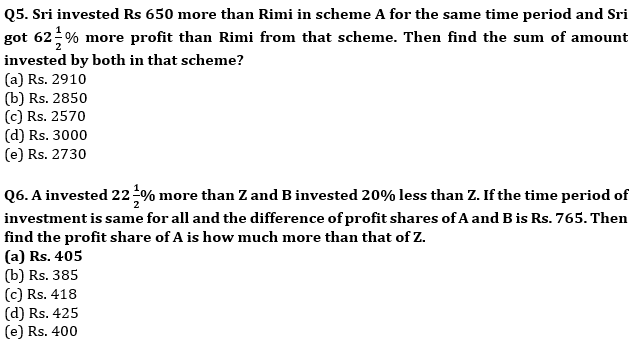Q7. Sumit’s investment is 1.5 times of the investment of Sahil and ratio between period of their investments is 2 : 1. If share of Sumit in total profit is Rs.7550 more than the share of Sahil in total profit, then find the total profit.
(a) Rs.7550
(b) Rs.15100
(c) Rs.14100
(d) Rs.13500
(e) Rs.15000

Q8. A bottle contains three-fourths of milk and the rest water. How much of the mixture must be taken away and replaced by an equal quantity of water so that the mixture has half milk and half water ?
(a) 25%
(b) 33.33%
(c) 45%
(d) 50%
(e) 66.67%

Q9. A chemist has 10 litre of a solution that is 10% nitric acid by volume. He wants to dilute the solution to 4% strength by adding water how many litre of water must be added?
(a) 15
(b) 20
(c) 18
(d) 25
(e) 17

Q10. A mixture of milk and water contains 60% milk and remaining water. How much water should be added (in percentage) in mixture to reverse the proportion of milk and water?
(a) 25%
(b) 37.5%
(c) 62.5%
(d) 75%
(e) 50%

Q11. A mixture of 25 ℓ contains milk and water in the ratio 3 : 2. ‘x’ ℓ of water is added in mixture to make the ratio of milk and water as 1 : 1. After that ‘y’ ℓ of milk is added to make the proportion of milk and water same as in initial condition. Find ‘y’ is what percent more than ‘x’ ?
(a) 12.5%
(b) 25%
(c) 37.5%
(d) 50%
(e) 62.5%

Q12. Fresh grapes contain 75% of water by weight while dried grapes contain 40% of pulp by weight. Find the weight of dry grapes obtained from 24 kg of fresh grapes?
(a) 10 kg
(b) 12 kg
(c) 15 kg
(d) 18 kg
(e) 20 kg

Q13. A container contained 60l milk. Out of this 6l of milk was taken out and replaced with water. This process was repeated two more times further. How much milk is now in container?(in litres)
(a) 43.74
(b) 42.74
(c) 44.74
(d) 41.74
(e) 45.74

Q14. A, B and C started a business with Rs60,000. Amount invested by ‘A and C’ together is twice than that of ‘B’ while amount invested by ‘A’ and ‘B’ together is thrice then that of ‘C’. ‘A’ invested for 6 months, ‘B’ for 9 months and ‘C’ for a year. Find the share of ‘B’ out of total profit of Rs 3400.(in Rs.)
(a) 1200
(b) 1800
(c) 1000
(d) 1400
(e) 1500

Q15. A mixture contains wine and water in the ratio 5 : 1. On adding 5 litre of water, the ratio of wine to water becomes 5 : 2. The quantity of wine in the mixture is ?
(a) 20 l
(b) 22 l
(c) 24 l
(d) 26 l
(e) 25 l

Practice More Questions of Quantitative Aptitude for Competitive Exams:

###### SBI Clerk Study Plan 2021

Solutions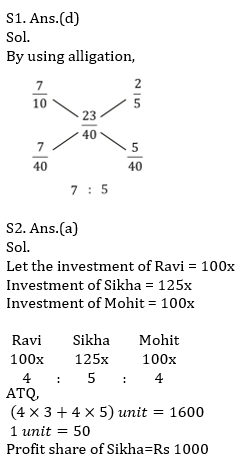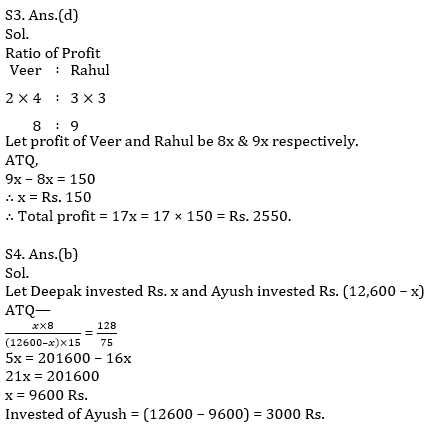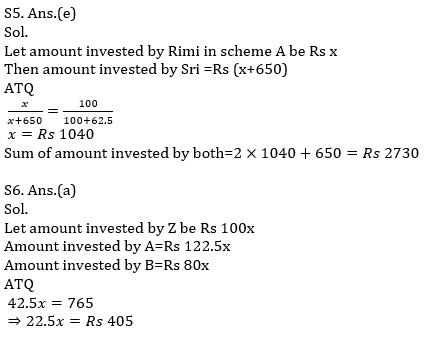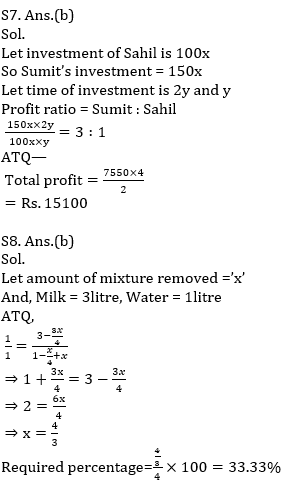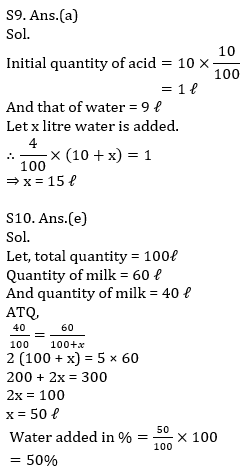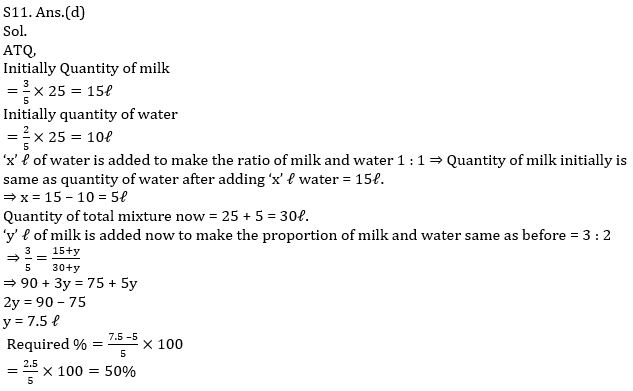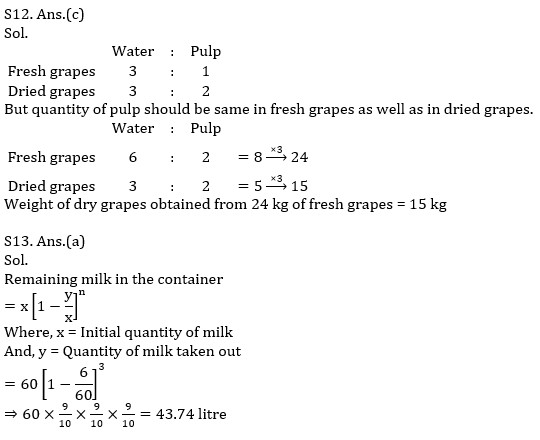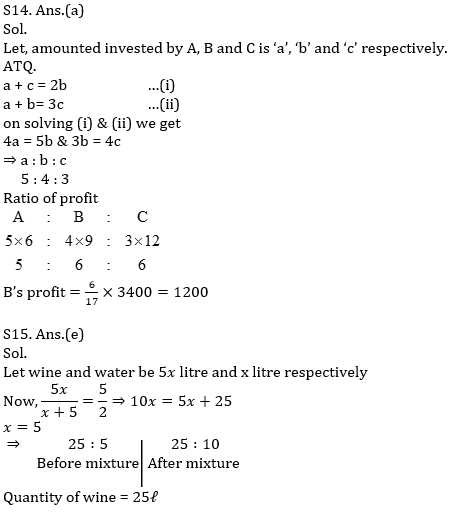#### Congratulations!Incorrect details? Fill the form again here

•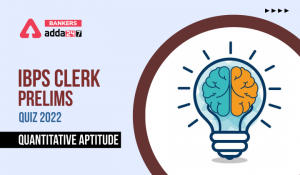Quantitative Aptitude Quiz For IBPS Cler...
•Quantitative Aptitude Quiz For IBPS RRB ...
•Quantitative Aptitude Quiz For IBPS RRB ...
•Quantitative Aptitude Quiz For IBPS Cler...
•Quantitative Aptitude Quiz For IBPS RRB ...
•Quantitative Aptitude Quiz For IDBI AM/E...